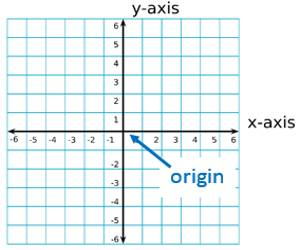The subject co-ordinate geometry is that particular branch of mathematics in which geometry is studied with the help of algebra. There are all sorts of ways that we can find the measurements of lines and angles. We can use rulers to measure lines and protractors to measure angles. This branch of mathematics was first introduced by the great French Philosopher and Mathematician Rene’ Descartes and by his name the subject is also called Cartesian Co-ordinate Geometry.

In coordinate geometry, we can use graphs and coordinates to find measurements and other useful information about geometric figures. In this geometry, the concept of algebra is introduced and as a result, the fundamental properties and theorems of geometry can be easily deduced. For this reason, sometimes this branch is called Analytical Geometry.Coordinate Geometry is of two types:

(i) Two Dimensional or Plane Co-ordinate Geometry

(ii) Three Dimensional or Solid Co-ordinate Geometry.

Co-ordinate Geometry is a system of geometry where the position of points on the plane is described using an ordered pair of numbers. Straight lines in coordinate geometry are the same idea as in regular geometry, except that they are drawn on a coordinate plane and we can do more with them.

In two-dimensional co-ordinate geometry, the discussion of geometry on a plane is developed whereas in three-dimensional co-ordinate geometry we consider the geometry of space of a solid body. The position of a point in two-dimensional co-ordinate geometry is uniquely determined by two real numbers with appropriate signs in three-dimensional Co-ordinate Geometry the position of a point on a solid body is uniquely determined by three sign real numbers.

Information Source: